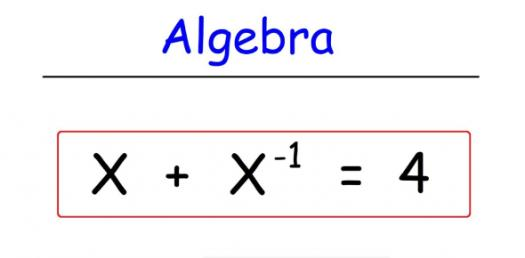# Algebra: Finding Equations Of Vertical And Horizontal Lines

3 Questions | Total Attempts: 527SettingsDo you know how to locate equations of vertical and vertical lines? Do you know how to read line graphs? A vertical line is one that goes straight up and down, parallel to the y-axis of the coordinate plane. Did you know that in mathematics, a vertical line test is a visual way to see if the curve is a graph of a function? Take this quiz! Prepare to learn!

Related Topics
• 1.
What is the equation of a vertical line going through (8,0)?
• A.

X =0

• B.

Y =0

• C.

X =8

• D.

Y =8

• 2.
Find the equation of the line going through the point (5,3) and has a slope of 0.
• 3.
What is the equation of the line graphed?